# Using Scientific Calculators: College Precalculus Lesson Plans Chapter Exam

Exam Instructions:

Choose your answers to the questions and click 'Next' to see the next set of questions. You can skip questions if you would like and come back to them later with the yellow "Go To First Skipped Question" button. When you have completed the practice exam, a green submit button will appear. Click it to see your results. Good luck!

### Page 1

#### Question 4 4. For these questions, I strongly recommend using either the CLEP calculator (found online) or one you use in class. What is the root of f(x)? (where f(x) is equal to zero)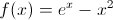### Page 2

#### Question 6 6. For these questions, I strongly recommend using either the CLEP calculator (found online) or one you use in class. Find x.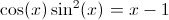#### Question 7 7. For these questions, I strongly recommend using either the CLEP calculator (found online) or one you use in class. Find x.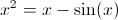#### Question 9 9. The following graph is which of the functions below?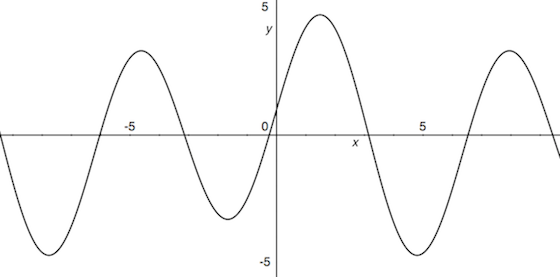#### Question 10 10. For these questions, I strongly recommend using either the CLEP calculator (found online) or one you use in class. Find x.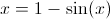### Page 3

#### Question 14 14. For these questions, I strongly recommend using either the CLEP calculator (found online) or one you use in class. What is the value of x where the following two lines intersect?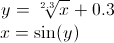#### Question 15 15. For these questions, I strongly recommend using either the CLEP calculator (found online) or one you use in class. Find x.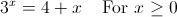### Page 4

#### Question 17 17. For these questions, I strongly recommend using either the CLEP calculator (found online) or one you use in class. Find x.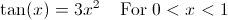#### Question 19 19. For these questions, I strongly recommend using either the CLEP calculator (found online) or one you use in class. Where is the maximum value of f(x) between 0 and pi?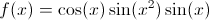#### Question 20 20. For these questions, I strongly recommend using either the CLEP calculator (found online) or one you use in class. At what is the minimum value of f(x) between 0 and 1?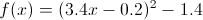#### Using Scientific Calculators: College Precalculus Lesson Plans Chapter Exam Instructions

Choose your answers to the questions and click 'Next' to see the next set of questions. You can skip questions if you would like and come back to them later with the yellow "Go To First Skipped Question" button. When you have completed the practice exam, a green submit button will appear. Click it to see your results. Good luck!

Support